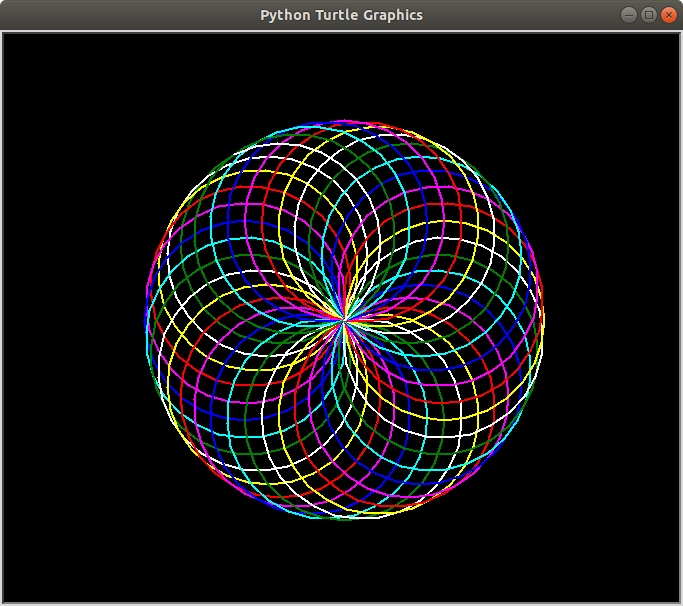Open in App
Not now

# Print a Spirograph using turtle in Python

• Difficulty Level : Basic
• Last Updated : 11 Sep, 2021

Pre-requisites: Turtle Programming in Python

A spirograph is a very interesting geometrical figure which is often symmetrical to both the axes. It produces mathematical roulette curves of the variety technically known as hypotrochoids and epitrochoids. Here, we’ve used a range of colors to draw circles, you can use your combination as per your color choice.

Below is the implementation.

## Python3

 `# Import the turtle library for ` `# drawing the required curve` `import` `turtle as tt`   `# Set the background color as black,` `# pensize as 2 and speed of drawing ` `# curve as 10(relative)` `tt.bgcolor(``'black'``)` `tt.pensize(``2``)` `tt.speed(``10``)`   `# Iterate six times in total` `for` `i ``in` `range``(``6``):` `  `  `      ``# Choose your color combination` `    ``for` `color ``in` `(``'red'``, ``'magenta'``, ``'blue'``, ` `                  ``'cyan'``, ``'green'``, ``'white'``,` `                  ``'yellow'``):` `        ``tt.color(color)` `        `  `        ``# Draw a circle of chosen size, 100 here` `        ``tt.circle(``100``)` `        `  `        ``# Move 10 pixels left to draw another circle` `        ``tt.left(``10``)` `    `  `    ``# Hide the cursor(or turtle) which drew the circle` `    ``tt.hideturtle()`

Output:My Personal Notes arrow_drop_up
Related Articles